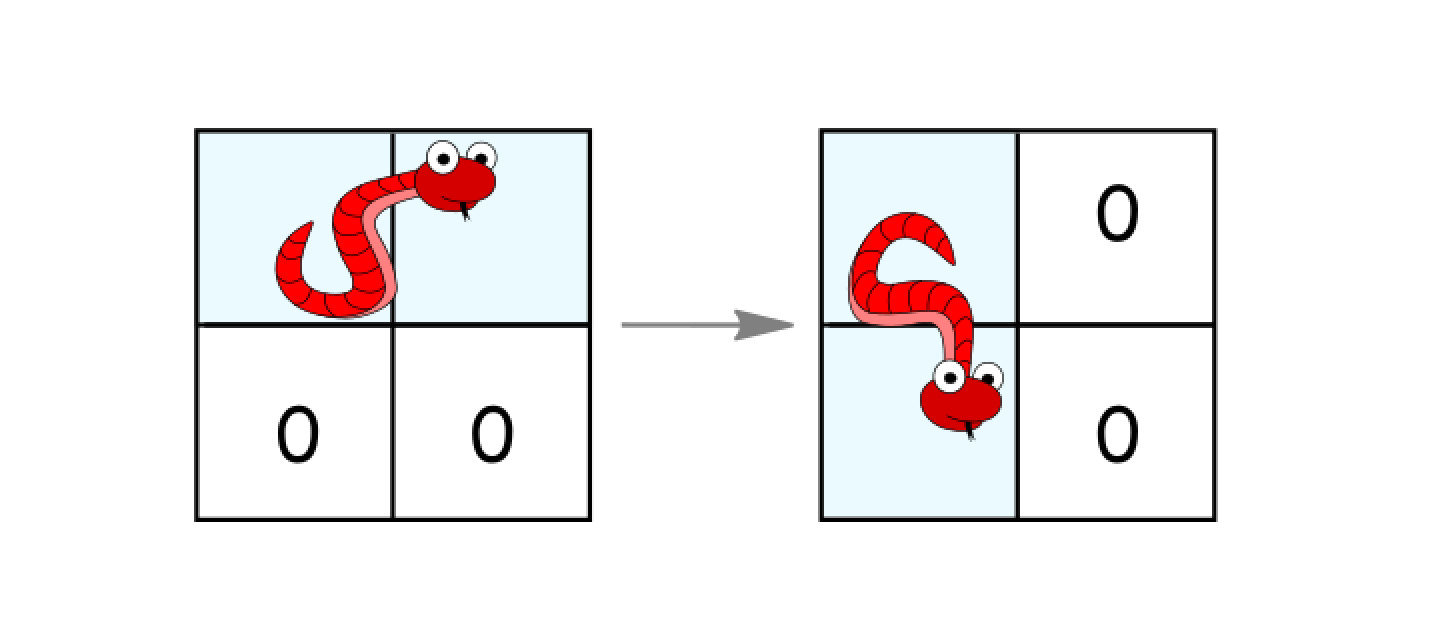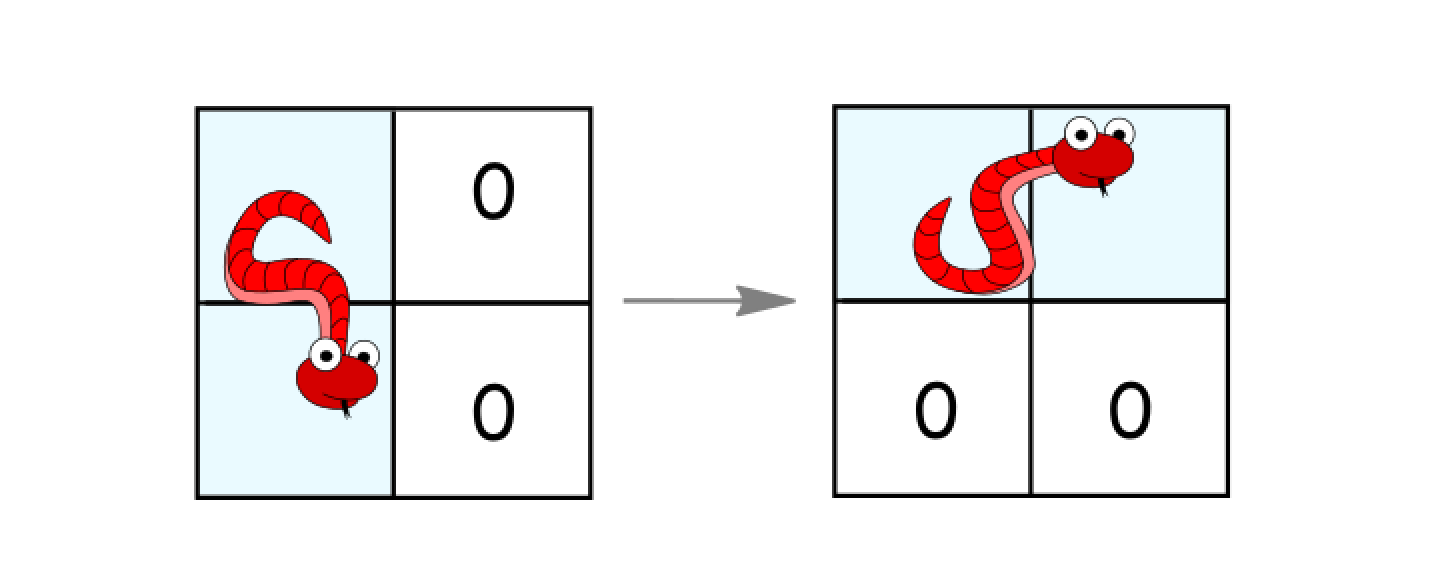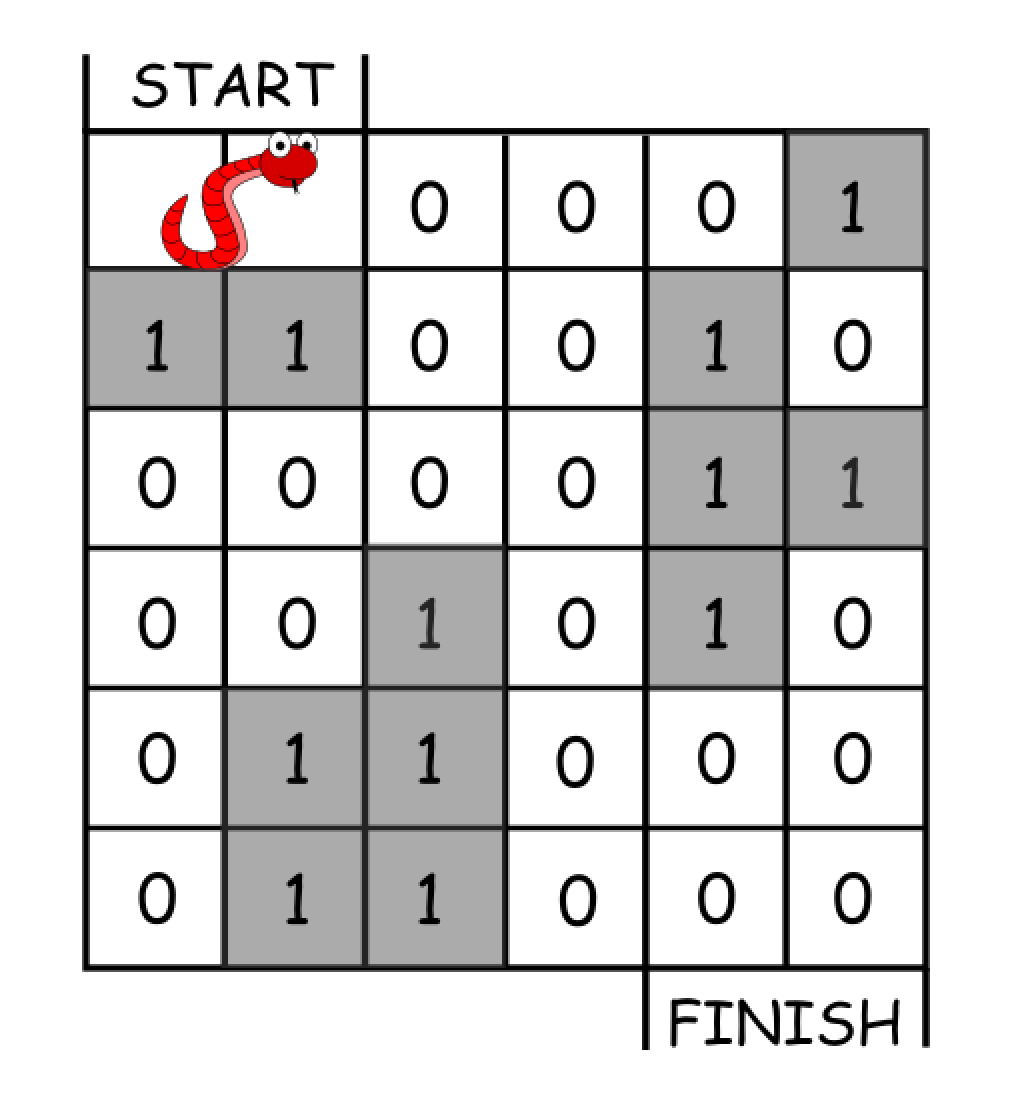# GeetCode Hub

In an `n*n` grid, there is a snake that spans 2 cells and starts moving from the top left corner at `(0, 0)` and `(0, 1)`. The grid has empty cells represented by zeros and blocked cells represented by ones. The snake wants to reach the lower right corner at `(n-1, n-2)` and `(n-1, n-1)`.

In one move the snake can:

• Move one cell to the right if there are no blocked cells there. This move keeps the horizontal/vertical position of the snake as it is.
• Move down one cell if there are no blocked cells there. This move keeps the horizontal/vertical position of the snake as it is.
• Rotate clockwise if it's in a horizontal position and the two cells under it are both empty. In that case the snake moves from `(r, c)` and `(r, c+1)` to `(r, c)` and `(r+1, c)`.• Rotate counterclockwise if it's in a vertical position and the two cells to its right are both empty. In that case the snake moves from `(r, c)` and `(r+1, c)` to `(r, c)` and `(r, c+1)`.Return the minimum number of moves to reach the target.

If there is no way to reach the target, return `-1`.

Example 1:```Input: grid = [[0,0,0,0,0,1],
[1,1,0,0,1,0],
[0,0,0,0,1,1],
[0,0,1,0,1,0],
[0,1,1,0,0,0],
[0,1,1,0,0,0]]
Output: 11
Explanation:
One possible solution is [right, right, rotate clockwise, right, down, down, down, down, rotate counterclockwise, right, down].
```

Example 2:

```Input: grid = [[0,0,1,1,1,1],
[0,0,0,0,1,1],
[1,1,0,0,0,1],
[1,1,1,0,0,1],
[1,1,1,0,0,1],
[1,1,1,0,0,0]]
Output: 9
```

Constraints:

• `2 <= n <= 100`
• `0 <= grid[i][j] <= 1`
• It is guaranteed that the snake starts at empty cells.

class Solution { public int minimumMoves(int[][] grid) { } }